#0
0
01. 云栖社区>
2. 博客>
3. 正文

## 初识推荐系统

### 存在问题（背景）

• 关键词的信息量不足，基于关键词的检索在很多情况下不能精准和深刻地反映用户的潜在需求；
• 通用的搜索引擎只有而且必须对用户提供尽量丰富而无差别的信息，这样才能应对不同种类的需求，以及需求的变换。

• 需求的定位；
• 需求的个性化；
• 需求的模糊性衍生。

### 推荐系统的应用

• 把包销售：经常一起购买的产品；
• 协同过滤：购买了此产品的顾客同时也购买的产品；（除了促销之外，也可以帮助用户定位购买需求）
• 用户的商品评论列表。

### 推荐系统的架构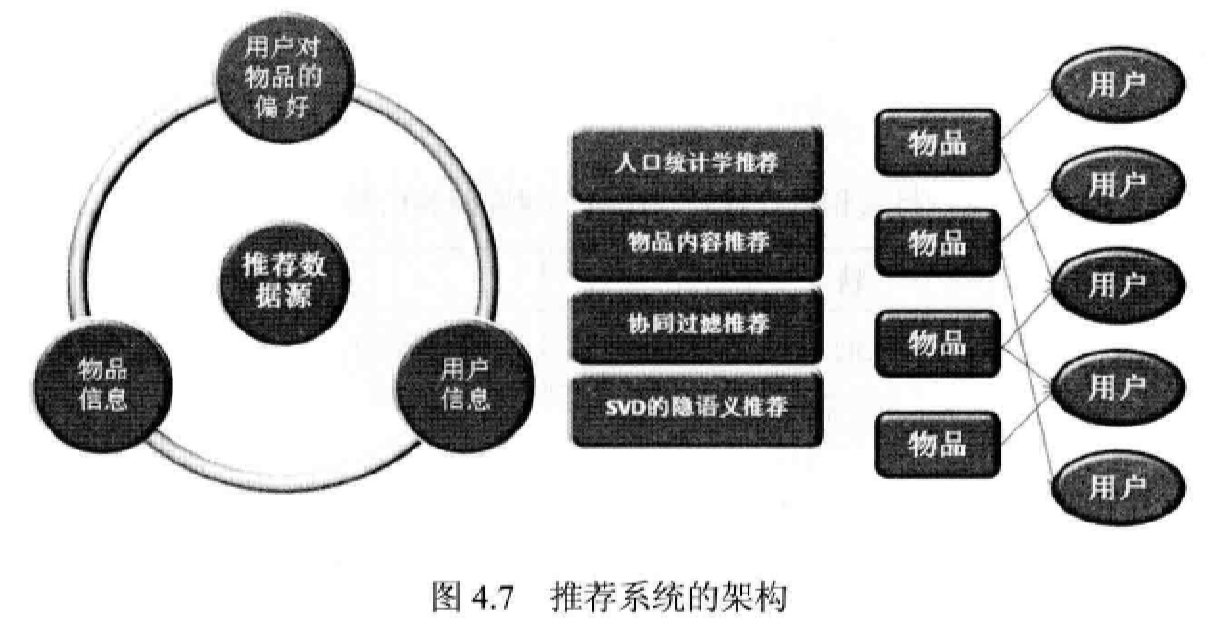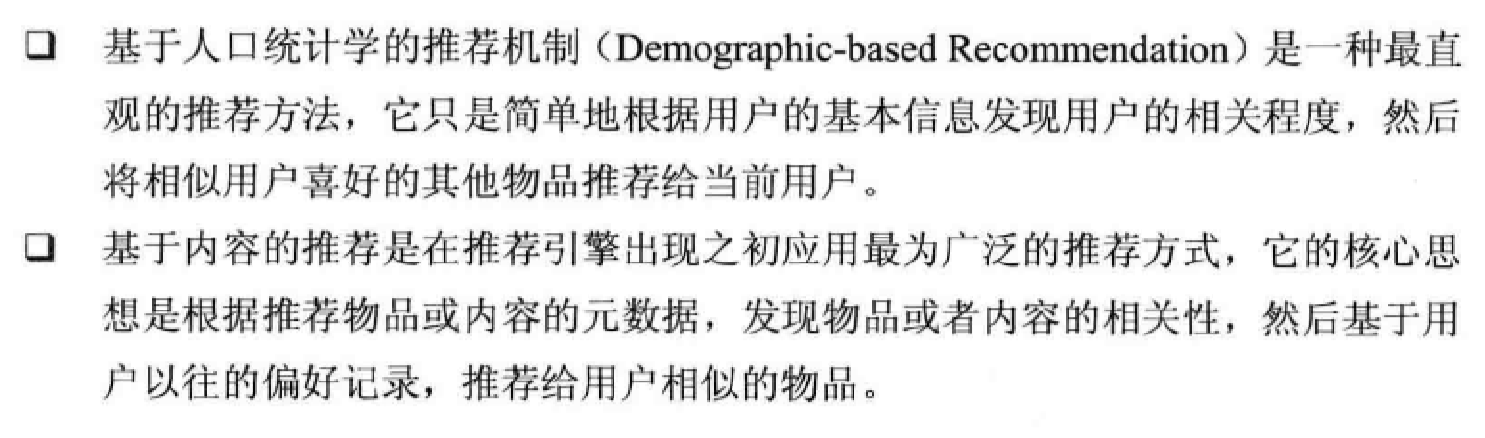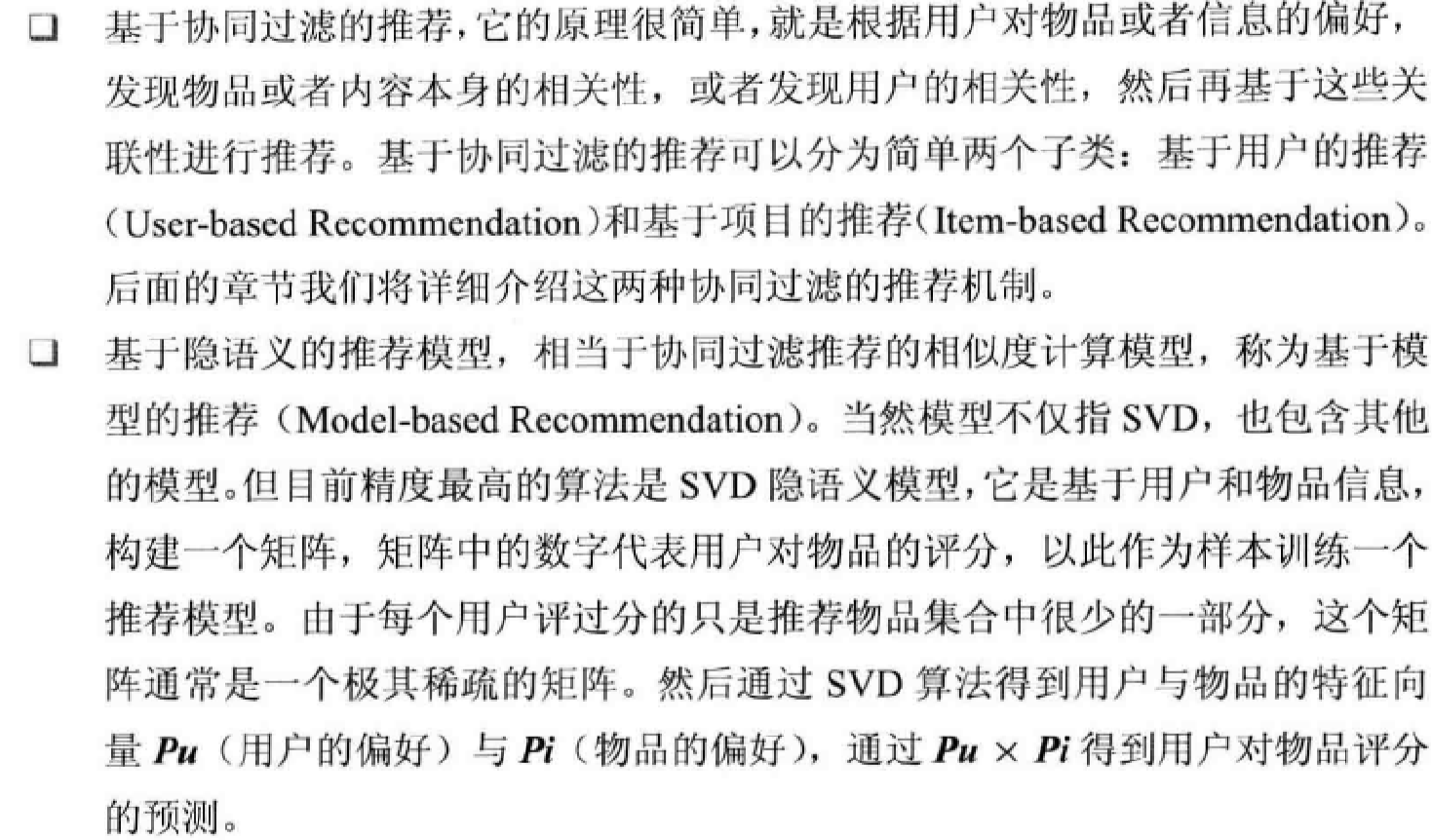## 协同过滤（Collaborative Filtering，CF）

• 基于用户的推荐技术：找到具有相似品味的人所喜欢的物品——User CF；
• 基于物品的推荐技术：从一个人喜欢的物品中找出相似的物品——Item CF。

### 数据预处理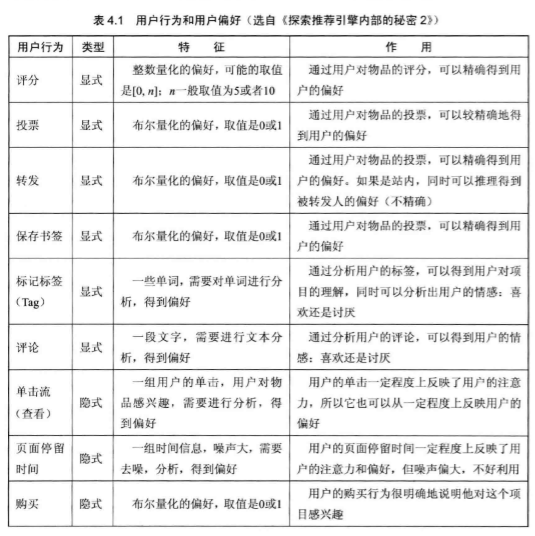• 减噪
• 归一化
• 聚类（缩减计算量）

### 使用 Scikit-Learn 的 KMeans 聚类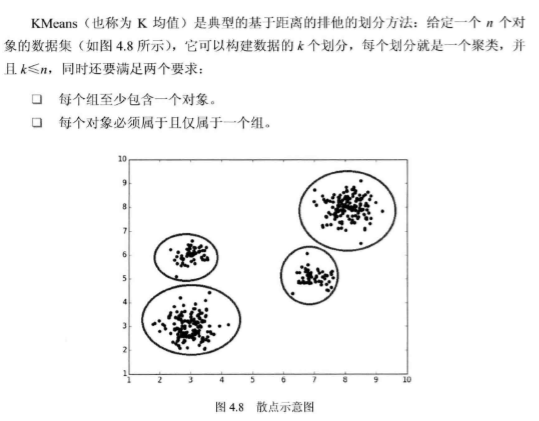### KMeans 的基本原理

• 首先创建一个初始划分，随机选择 \(k\) 个对象，每个对象初始地代表了一个聚类中心。对于其他对象，根据其与各个聚类中心的距离，将它们赋给最近的簇。
• 然后采用一种迭代的重定位技术，尝试通过对象在划分的簇之间移动来改进划分，直到聚类中心不发生变化为止。
• 重定位技术：就是当有新的对象加入到簇中或已有对象离开簇时，重新计算聚类的平均值（作为聚类中心），然后对对象进行重新分配。
``````import os
import pandas as pd

import sys
sys.path.append('E:/xinlib')
import chaos

root = 'D:/MLBook' + '/chapter04/testdata'
os.listdir(root)``````
``````['4k2_far.txt',
'figure_0.png',
'figure_1.png',
'figure_2.png',
'figure_3.png',
'testSet.txt']``````

`File2Table` 的使用参考：机器学习的数学基础

``````T = chaos.File2Table(root)
for p in T.to_pandas('\t'):
break``````
``p.head()``
0 1 2
0 1 2.7266 3.0102
1 1 3.1304 2.4673
2 1 3.0492 2.525
3 1 3.226 3.1649
4 1 2.7223 2.5713

``p.dtypes``
``````0    object
1    object
2    object
dtype: object``````
``````p[[1, 2]] = p[[1, 2]].astype(float)
p[] = p[].astype(int)
p.dtypes``````
``````0      int32
1    float64
2    float64
dtype: object``````
``````from sklearn.cluster import KMeans
from pylab import mpl

mpl.rcParams['font.sans-serif'] = ['FangSong'] # 指定默认字体
mpl.rcParams['axes.unicode_minus'] = False # 解决保存图像是负号'-'显示为方块的问题

%pylab inline``````
``Populating the interactive namespace from numpy and matplotlib``
``````k = len(set(p))
M = p[[1, 2]]
kmeans = KMeans(init='k-means++', n_clusters=k)
kmeans.fit(M)``````
``````KMeans(algorithm='auto', copy_x=True, init='k-means++', max_iter=300,
n_clusters=4, n_init=10, n_jobs=1, precompute_distances='auto',
random_state=None, tol=0.0001, verbose=0)``````
``````ax = p.plot.scatter(x=1, y=0, color='DarkRed', label='feture1')
# 将之下这个 data 画在上一个 ax 上面
p.plot.scatter(x=2, y=0, color='LightGreen', label='feture2', ax=ax)
plt.show()``````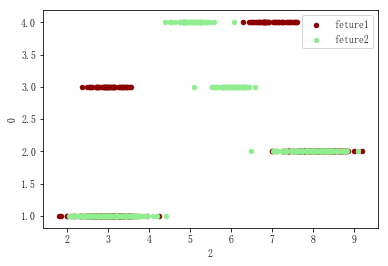``````cluster_centers = pd.DataFrame(kmeans.cluster_centers_, columns=['cluster_centers_1', 'cluster_centers_2'])
cluster_centers``````
cluster_centers_1 cluster_centers_2
0 3.022117 6.007702
1 8.081695 7.975067
2 2.958321 2.985985
3 6.994380 5.054563
``````ax = p.plot.scatter(x=1, y=2, color='DarkBlue', label='数据', s=20)
cluster_centers.plot.scatter(x='cluster_centers_1', y='cluster_centers_2', color='LightGreen', label='聚类中心', ax=ax, s=70)
plt.show()``````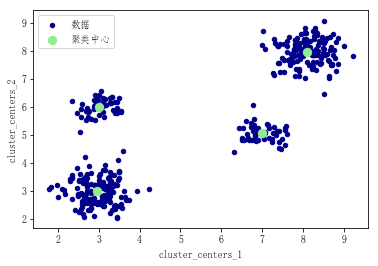+ 关注